### Distributions

The distributions,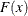, allowed in the PROBIT procedure are specified with the DISTRIBUTION= option in the MODEL statement. The cumulative distribution functions for the available distributions are

Cumulative Distribution Function

Distribution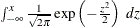Normal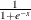Logistic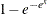Extreme value or Gompertz

The variances of these three distributions are not all equal to 1, and their means are not all equal to zero. Their means and variances are shown in the following table, whereis the Euler constant.

Distribution

Mean

Variance

Normal

0

1

Logistic

0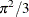Extreme value or Gompertz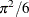When comparing parameter estimates by using different distributions, you need to take into account the different scalings and, for the extreme value (or Gompertz) distribution, a possible shift in location. For example, if the fitted probabilities are in the neighborhood of 0.1 to 0.9, then the parameter estimates from the logistic model should be about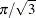larger than the estimates from the probit model.